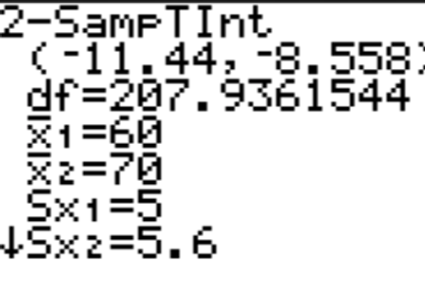## Day 106 - Lesson 10.2

##### Learning Targets
• Determine whether the conditions are met for doing inference about a difference between two means.

• Construct and interpret a confidence interval for a difference between two means.

##### Activity:

Be sure to buy cookies the day before this lesson – one package of Chips Ahoy and one package of store brand (in Michigan we have Meijers!). Each pair of students will get 1 Chips Ahoy cookie and 1 store brand cookie.

**Tip: It doesn’t matter how the chips are counted as long as students are consistent with both cookies. Counting the visible chips is easiest, but you could be like this student and disect them cookies.**

Students have all of the pieces of the puzzle to create this confidence interval without the help of the teacher. They know the four step process. They know how to construct a confidence interval. They know the formulas for the sampling distribution of the difference of means. Here they put it all together. Be sure that students clearly show the direction of their subtraction (Chips Ahoy – Store Brand). The last question of the Activity previews significance tests (which students will do tomorrow) by asking if the confidence interval provides convincing evidence that there is a difference in the average number of chocolate chips between the two types of cookies. The answer will depend on whether or not the confidence interval contains 0 (it is plausible that the two types of cookies are the same) or does not contain 0 (we have convincing evidence of a difference).##### HELP! Degrees of Freedom

The only piece of information that is missing for students to be able to construct this confidence interval is the degrees of freedom. Tell them it is easy…just use this formula:OK we lied. Not so easy. But it is easy to put the data into two lists on your TI-83/84 calculator and then do a 2-SampTInt or 2-SampTTest.Steal the degrees of freedom from the calculator. Then take this df to Table B to get a t* value (remember to round down the df if you are between values). Because students might be rounding down their df to get a t* value, their interval could be slightly wider than the one provided by the calculator. This is OK and students should not just write down the interval from the calculator – they must make sure that their interval matches with the t* value they recorded in their work.

##### Should I Pool?

If you are at the Holiday Inn, yes. If you are doing a two-sample t interval, no. We would only “pool” if we had reason to believe the the standard deviation was the same from both populations of cookies. AP Statistics students will never have to pool for two-sample t-procedures. Note: this is a different idea than the p-pooled or p-combined from a two-sample z test for the difference of proportions. In that case, we do “pool” or “combine” the data because we assumed the null hypothesis is true (p1=p2).